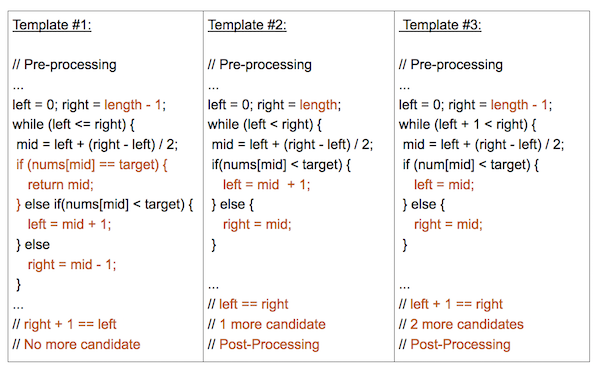Binary search is a search algorithm that finds the position of a target element in a sorted array. Binary search is much faster than linear search (yes, that search algorithm you use most of in your code).

Well, saying much faster means nothing - but there is a mathematical proof for that and it’s called Big O. Big O is a mathematical notation that tells us how our algorithm performs. We can separate this into 2 sections:

• Time complexity
• Space complexity

Time complexity tells us how fast is our algorithm and Space complexity, as you can guess, it’s about how much memory it will take.

If we measure time complexity in linear search we would get O(n) -> this is the worst-case scenario where if we have 10 records, a worst-case is that the answer is 10. Binary search however, has an O(logn), in a worst-case scenario, for example, n = 10, it will take logn10 which is ~3. This tells us, that binary search in the worst case will take 3 steps while linear search would take 10 for n = 10.

I’m not a mathematical genius, so let’s write binary search in go and benchmark it with the linear search!Binary Search

``````func BinarySearch(nums []int, target int) int {
left := 0
right := len(nums) - 1

for left <= right {
mid := left + (right-left)/2

if nums[mid] == target {
return mid
}

if nums[mid] > target {
right = mid - 1
} else {
left = mid + 1
}
}
return -1
}
``````

Our lovely linear search :)

``````func LinearSearch(nums []int, target int) int {
for i := range nums {
if nums[i] == target {
return i
}
}
return -1
}
``````

Before going into the benchmark, let’s first discuss the code for binary search. Hopefully, no explanation is needed for Linear Search :)

To understand how it works, lets split it into steps:

• Step 1: start by creating the `left` and `right` indexes.
• Step 2: go into loop until `left` <= `right`.
• Step 3: find a middle position `mid`. In this case it’s `left + (right - left) / 2`. Warning, it’s not `(left + right) / 2` because of the overflow.
• Step 4 - if `nums[mid]` is equal to `target`, we found the index! Return
• If `target` is not found, repeat the following:
• If `nums[mid]` is greater than the target, we know that our `target` is not on the `right` side, so we close the gap by doing `right = mid - 1`
• If `nums[mid]` is less than the target, we know that our `target` is somewhere at the `left` side, so we close the gap by doing `left = mid + 1`
• If we exit the loop, `target` is not found and we return -1 or whatever that tells us that the index was not found

Make sense?

Ok, good, now let’s do the benchmark. In this benchmark, we will create 1,000,000 records (which are sorted) and perform a binary and linear search. Results?

goos: linux

goarch: amd64

cpu: AMD Ryzen 9 5900X 12-Core Processor

BenchmarkBinarySearch-24 43950141 27.83 ns/op

BenchmarkLinearSearch-24 873769 112537 ns/op

We can easily see who is a clear winner here. Does that mean you should be using binary search on sorted data? Well, not always. Do you remember that I was talking about the worst case? What about the best case? If we set to find number 1, who would win?

goos: linux

goarch: amd64

cpu: AMD Ryzen 9 5900X 12-Core Processor

BenchmarkBinarySearch-24 44865939 26.01 ns/op

BenchmarkLinearSearch-24 581687065 2.055 ns/op

Suprise, linear search wins! :)

A rule of thumb

If you need to find a value in a large sorted dataset:

• And you are sure that the value will always be somewhere at the start, use a linear search.
• Use binary search
• And not sure which search algorithm to use, use binary search

This algorithm can be transformed to solve many problems, not just search for value.

Example: Let’s say you get a number and your favorite language does not have a math library to get a square root of that number. How would you get a square root? A simple brute-force, linear type of thing will work:

``````func FindSquareRoot(num int) int {
// if we get 16, we know that square root is somewhere between 2 and 8
for i := 2; i < num/2; i++ {
if i*i == num {
return i
}
}
return -1
}
``````

Can we modify our binary search to support this?

``````func FindSquareRoot(num int) int {
left := 2
right := num / 2
for left <= right {
mid := left + (right-left)/2
if mid*mid == num {
return mid
}
if mid*mid > num {
right = mid - 1
} else {
left = mid + 1
}
}

return -1
}
``````

There are also some base cases missing, can you spot them? (what is the square root of 1? :) )

Binary search can go into different shapes and forms, so don’t discard it if you don’t have sorted data - try to brute-force it first and think about optimization. Can it be optimized with binary search?

There are 3 templates of binary search that can be used to solve problems:Happy coding!